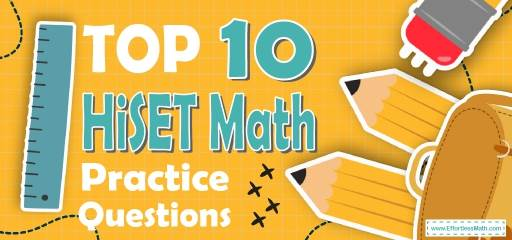# Top 10 HiSET Math Practice QuestionsPreparing for the HiSET Math test? The best way to prepare for your HiSET Math test is to work through as many HiSET Math practice questions as possible. Here are the top 10 HiSET Math practice questions to help you review the most important HiSET Math topics. These HiSET Math practice questions are designed to cover mathematics concepts and topics that are found on the actual test. The questions have been fully updated to reflect the latest 2022 HiSET guidelines. Answers and full explanations are provided at the end of the post.

Start your HiSET Math test prep journey right now with these samples of HiSET Math questions.

## HiSET Math Practice Questions

1- When a number is subtracted from $$24$$ and the difference is divided by that number, the result is $$3$$. What is the value of the number?

A. $$2$$

B. $$4$$

C. $$6$$

D. $$12$$

E. $$24$$

2- An angle is equal to one-fifth of its supplement. What is the measure of that angle?

A. $$20$$

B. $$30$$

C. $$45$$

D. $$60$$

E. $$90$$

3- John traveled 150 km in 6 hours and Alice traveled 180 km in 4 hours. What is the ratio of the average speed of John to the average speed of Alice?

A. $$3:2$$

B. $$2:3$$

C. $$5:9$$

D. $$5:6$$

E. $$11:16$$

4- If $$40\%$$ of a class are girls, and $$25\%$$ of girls play tennis, what percent of the class play tennis?

A. $$10 \%$$

B. $$15 \%$$

C. $$20 \%$$

D. $$40 \%$$

E. $$80 \%$$

5- What is the value of $$y$$ in the following system of equation?
$$3x-4y= -20$$
$$-x+2y= 10$$

A. $$-1$$

B. $$-2$$

C. $$1$$

D. $$4$$

E. $$5$$

6- In five successive hours, a car travels 40 km, 45 km, 50 km, 35 km, and 55 km. In the next five hours, it travels with an average speed of 50 km per hour. Find the total distance the car traveled in 10 hours.

A. 425 km

B. 450 km

C. 475 km

D. 500 km

E. 1,000 km

7- How long does a 420–miles trip take moving at 50 miles per hour (mph)?

A. 4 hours

B. 6 hours and 24 minutes

C. 8 hours and 24 minutes

D. 8 hours and 30 minutes

E. 10 hours and 30 minutes

8- What is the difference between the smallest 4–digit number and the biggest 4–digit number?

A. 6666

B. 6789

C. 8888

D. 8999

E. 9999

9- What is the value of $$6^4$$?

A. 6

B. 24

C. 36

D. 216

E. 1,296

10- Right triangle ABC has two legs of lengths 6 cm (AB) and 8 cm (AC). What is the length of the third side (BC)?

A. 4 cm

B. 6 cm

C. 8 cm

D. 10 cm

E. 20 cm

## Best HiSET Math Prep Resource for 2022

1- C
Let $$x$$ be the number. Write the equation and solve for $$x$$.
$$(24 – x) ÷ x = 3$$
Multiply both sides by $$x$$.
$$(24 – x) = 3x$$, then add $$x$$ both sides. $$24 = 4x$$, now divide both sides by $$4$$.
$$x = 6$$

2- B
The sum of supplement angles is $$180$$. Let $$x$$ be that angle. Therefore, $$x + 5x = 180$$
$$6x = 180$$, divide both sides by $$6: x = 30$$

3- C
The average speed of john is: $$150 ÷ 6 = 25$$
The average speed of Alice is: $$180 ÷ 4 = 45$$
Write the ratio and simplify.
$$25 : 45 ⇒ 5 : 9$$

4- A
The percent of girls playing tennis is: $$40 \% × 25 \% = 0.40 × 0.25 = 0.10 = 10 \%$$

5- E
Solving Systems of Equations by Elimination
$$3x-4y= -20$$
$$-x+2y=10$$
Multiply the second equation by $$3$$, then add it to the first equation.
$$3x-4y= -20$$
$$3(-x+2y=10)$$
$$\Downarrow$$
$$3x-4y= -20$$
$$-3x+6y=30$$
$$\Downarrow$$
$$2y= 10$$
$$\Downarrow$$
$$y= 5$$

6- C
Add the first $$5$$ numbers. $$40 + 45 + 50 + 35 + 55 = 225$$
To find the distance traveled in the next $$5$$ hours, multiply the average by number of hours.
Distance = Average $$×$$ Rate $$= 50 × 5 = 250$$
Add both numbers. $$250 + 225 = 475$$

7- C
Use distance formula:
Distance = Rate $$×$$ time $$⇒ 420 = 50 ×$$ T, divide both sides by $$50$$. $$420 \div 50 = T ⇒ T = 8.4$$ hours.
Change hours to minutes for the decimal part. $$0.4$$ hours $$= 0.4 × 60 = 24$$ minutes.

8- D
Smallest $$4$$–digit number is $$1000$$, and biggest $$4$$–digit number is $$9999$$. The difference is: $$8999$$.

9- E
$$6^4 = 6 × 6 × 6 × 6 = 1296$$

10- D
Use Pythagorean Theorem: $$a^2 + b^2 = c^2$$
$$6^2 + 8^2 = c^2 ⇒ 100 = c^2 ⇒ c = 10$$

## High School Equivalency Tests

Looking for the best resources to help you succeed on the HiSET Math test?

## The Best Books to Ace the HiSET Math Test

### What people say about "Top 10 HiSET Math Practice Questions - Effortless Math: We Help Students Learn to LOVE Mathematics"?

No one replied yet.

X
52% OFF

Limited time only!

Save Over 52%

SAVE $40 It was$76.99 now it is \$36.99